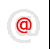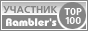Apache-Talk @lexa.ruInet-Admins @info.east.ruSecurity-alerts @yandex-team.runginx-ru @sysoev.ruđéűéôĺđéóříááňčé÷ :: Filmscanners
Filmscanners mailing list archive (filmscanners@halftone.co.uk)

### [filmscanners] Terms used in Dynamic Range calculations...

```Reference this diagram:

http://www.darkroom.com/Images/DynamicRange01.jpg

"largest" is shown on this diagram to be the maximum signal level minus the
minimum signal level, and is the largest range or absolute range that the
signal can go from <=> to.  Example, maximum signal level is 5, minimum
signal level is 2, the absolute range/largest range is (5 - 2) or 3.

"smallest" is shown as the noise.  It is the same thing as "smallest
discernable signal", which means the smallest change that can be detected.

Where the signal is at it's lowest point, is what I am calling "minimum
signal level".

Where the signal is at it's highest point, is what I am calling "maximum
signal level".

The dynamic range equation is, using these terms, and the provided diagram,
can be either of the following:

DR (dB) = 10log10 (largest/smallest)

DR (dB) = 10log10 ((maximum signal level - minimum signal level) / smallest)

You can substitute "smallest DISCERNABLE signal" for "smallest" in these
equations.  Do NOT confuse "smallest"/"smallest discernable signal" with
"minimum signal level", they are not the same thing, though in some
circumstances they MAY have the same value.

Now, if you are using log values for "largest" and "smallest", then you have
the following equation:

DR (dB) = largest(in dB) - smallest (in dB)

since subtraction in log is the same as division in non-log nomenclature.

The ISO spec states:

"DR = Dmax - Dmin"

Since density values are in log form, this is the correct equation.

The ISO spec defines:

"Dmax = Density where the Signal to noise ratio is 1"

Which is correct, as it is the lowest signal level that will come from the
scanner electronics when measuring the highest density, and where the signal
and noise will be equal.  This value obviously takes into account noise.
They make the assumption that the noise is the same as the "minimum signal
level", which is fine for the case they are using it for (more below).

"Dmin = Minimum density where the output signal of the luminance OECF
appears to be unclipped"

Again, correct, as that is when you get the lowest density, when you are
reading the strongest signal from the electronics.

Because this is in log form, subtraction is used...and therefore, noise can
be taken into account in EITHER of the terms.

Note (VERY important, and I believe a source of confusion, and read
CAREFULLY), they are talking about the dynamic range of the SCANNER.  If you
scan something with this scanner, obviously, since the image may have a
smaller density range than the scanner can accommodate, that image and
therefore the image data from the scanner will have a different DMax and
DMin than that of the scanner.  BUT the inherent noise in the scanner is the
same, no matter what image you scan (non-linearities aside, assuming a
linear noise throughout the range)...so the dynamic range of the image data
you get from the scan will NOT have the same dynamic range as that of the
scanner it self, the image dynamic range will be less, assuming the image
did not occupy the full density range of the scanner.  Because of this, the
"minimum signal level" (what they call Dmax) and noise are NOT the same, and
the equation then changes and is not the same as the one ISO uses, as they
make an assumption that is now not valid.

Austin

----------------------------------------------------------------------------------------
Unsubscribe by mail to listserver@halftone.co.uk, with 'unsubscribe
filmscanners'
or 'unsubscribe filmscanners_digest' (as appropriate) in the message title or
body

```Copyright © Lexa Software, 1996-2009.